Also: More animations
Next: The bifurcation diagram

# Boundary crisis in quasiperiodically forced systems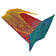This is a joint project with Ulrike Feudel, University of Potsdam (Germany). We study boundary crisis in quasiperiodically forced systems using the Hénon map as a characteristic example. The quasiperiodically forced Hénon map is defined as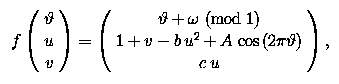with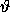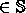1 and u, v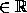. We consider the two-parameter family of maps in (A, b)-space where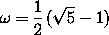is a fixed quasiperiodic rotation in, and c = 0.1.

A boundary crisis is the sudden disappearance of a chaotic attractor as it hits an unstable (periodic) set on its basin boundary. For example, if we take A = 0.2 the attractor becomes chaotic approximately for b = 0.896 and runs into an invariant circle of saddle type approximately for b = 1.334.An animated gif shows how the attractor for A = 0.2 changes as the parameter b increases from 0.5 to 1.35. The attractor hits the unstable invariant circle for b in between 1.33 and 1.34 (31KB).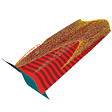Rotation about the u-axis shows how the attractor is about to touch its basin boundary. The boundary crisis is induced by the homoclinic tangency of the stable and unstable manifolds of the circle on the basin boundary. The parameters are A = 0.2 and b = 1.33 in this animation (943KB).

Also: More animations
Next: The bifurcation diagram